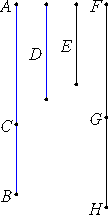# Proposition 53

To find the sixth binomial line.

Set out two numbers AC and CB such that AB does not have to either of them the ratio which a square number has to a square number, and let there also be another number D which is not square and which does not have to either of the numbers BA or AC the ratio which a square number has to a square number.Set out any rational straight line E, and let it be contrived that D is to AB as the square on E is to the square on FG. Then the square on E is commensurable with the square on FG. And E is rational, therefore FG is also rational.

X.9

Now, since D does not have to AB the ratio which a square number has to a square number, neither does the square on E have to the square on FG the ratio which a square number has to a square number, therefore E is incommensurable in length with FG.

Again, let it be contrived that BA is to AC as the square on FG is to the square on GH. Then the square on FG is commensurable with the square on HG.

X.9

Therefore the square on HG is rational. Therefore HG is rational. And, since BA does not have to AC the ratio which a square number has to a square number, neither does the square on FG have to the square on GH the ratio which a square number has to a square number, therefore FG is incommensurable in length with GH.

X.36

Therefore FG and GH are rational straight lines commensurable in square only. Therefore FH is binomial.

It is next to be proved that it is also a sixth binomial straight line.

V.22

Since D is to AB as the square on E is to the square on FG, and also BA is to AC as the square on FG is to the square on GH, therefore, ex aequali, D is to AC as the square on E is to the square on GH.

X.9

But D does not have to AC the ratio which a square number has to a square number, therefore neither does the square on E have to the square on GH the ratio which a square number has to a square number, therefore E is incommensurable in length with GH.

But it was also proved incommensurable with FG, therefore each of the straight lines FG and GH is incommensurable in length with E.

And, since BA is to AC as the square on FG is to the square on GH, therefore the square on FG is greater than the square on GH.

V.19,Cor.

Let then the sum of the squares on GH and K equal the square on FG. Then, in conversion, AB is to BC as the square on FG is to the square on K.

But AB does not have to BC the ratio which a square number has to a square number, so that neither does the square on FG have to the square on K the ratio which a square number has to a square number.

X.9

Therefore FG is incommensurable in length with A. Therefore the square on FG is greater than the square on GH by the square on a straight line incommensurable with FG.

And FG and GH are rational straight lines commensurable in square only, and neither of them is commensurable in length with the rational straight line E set out.

Therefore FH is a sixth binomial straight line.

Q.E.D.

## Guide

This proposition is not used in the rest of the Elements.